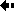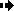## Effective population sizeEffective population size is the number of individuals in a population who contribute offspring to the next generation.

In an ecological sense, the size of a population can be measured by simply counting the number of adults in a locality. However, for the theory of population genetics what matters is the chance that two copies of a gene will be sampled as the next generation is produced, and this is affected by the breeding structure of the population.

In a population of size N there will be 2N genes. The correct interpretation of N for the theoretical equations is that N has been correctly measured when the chance of drawing two copies of the same gene is (1/2N)2. If we draw two genes from a population at a locality, we may be more likely for various reasons to get two copies of the same gene than would be implied by the naive ecological measure of population size.

Population geneticists therefore often write Ne (for 'effective' population size) in the equations, rather than N. In practice, effective population sizes are usually lower than ecologically observed population sizes.

What factors influence effective population size?Previous Next